# Abstracts

Multiple product inventories of forests require accurate estimates of the diameter, length and volume of each product. Taper functions have been used to precisely describe tree form, once they provide estimates for the diameter at any height or the height at any diameter. This study applied a goal programming technique to estimate the parameters of two taper functions to describe individual tree forms. The goal programming formulation generates parameters that minimize total absolute deviations (MOTAD). These parameters generated by the MOTAD method were compared to those of ordinary least squares (OLS) method. The analysis used a set of 178 trees cut from cloned eucalyptus plantations in the Southern part of the state of Bahia, Brazil. The values of the estimated parameters for the two taper functions resulted very similar when the two methods were compared. There was no significant difference between the two fitting methods according to the statistics used to evaluate the quality of the generated estimates. OLS and MOTAD resulted equally precise in the estimation of diameters and volumes outside and inside bark.

MOTAD; ordinary least squares; goal programming; linear regression

Os inventários florestais para múltiplos produtos requerem estimativas exatas do diâmetro, comprimento e volume de cada produto. As equações de afilamento têm sido usadas para descrever precisamente a forma da árvore uma vez que estas funções fornecem estimativas de diâmetro a qualquer altura ou de altura em qualquer diâmetro. Este trabalho aplica um modelo de programação por metas para estimar os parâmetros de duas equações de afilamento para descrever a forma do tronco de árvores individuais. O modelo de programação por metas gera parâmetros que minimizam a soma dos desvios absolutos (MOTAD). Esses parâmetros gerados pelo método MOTAD foram comparados aos parâmetros gerados pelo método dos mínimos quadrados ordinários (OLS). A análise se baseou em dados de cubagem de 178 árvores obtidas em plantios clonais de eucaliptos conduzidos na região sul da Bahia. Os valores dos parâmetros estimados por ambos os métodos de ajuste para as duas funções de afilamento mostraram-se muito semelhantes. Não houve diferença significativa entre os indicadores usados para avaliar a qualidade dos parâmetros estimados pelos dois métodos de ajuste. Os métodos OLS e MOTAD mostraram-se igualmente precisos na estimação de diâmetros e volumes com casca e sem casca.

minimização do desvio absoluto total; mínimos quadrados ordinários; programação por metas; regressão linear

FORESTRY SCIENCE

Fitting a taper function to minimize the sum of absolute deviations

Ajuste de uma função de afilamento via minimização da soma dos desvios absolutos

Lana Mirian Santos da SilvaI; Luiz Carlos Estraviz RodriguezII, * * Corresponding author < lcer@esalq.usp.br> ; José Vicente Caixeta FilhoIII; Simone Carolina BauchIV

IAthena Recursos Naturais - R. Treze de Maio, 768 - sala 21 - 13400-300 - Piracicaba, SP - Brasil

IIUSP/ESALQ - Depto. de Ciências Florestais, C.P. 09 - 13418-900 - Piracicaba, SP - Brasil

IIIUSP/ESALQ - Depto. de Economia, Administração e Sociologia

IVIMAZON - Instituto do Homem e Meio Ambiente da Amazônia - Rua Domingos Marreiros, 2020, 66060-160 - Belém, PA - Brasil

ABSTRACT

Multiple product inventories of forests require accurate estimates of the diameter, length and volume of each product. Taper functions have been used to precisely describe tree form, once they provide estimates for the diameter at any height or the height at any diameter. This study applied a goal programming technique to estimate the parameters of two taper functions to describe individual tree forms. The goal programming formulation generates parameters that minimize total absolute deviations (MOTAD). These parameters generated by the MOTAD method were compared to those of ordinary least squares (OLS) method. The analysis used a set of 178 trees cut from cloned eucalyptus plantations in the Southern part of the state of Bahia, Brazil. The values of the estimated parameters for the two taper functions resulted very similar when the two methods were compared. There was no significant difference between the two fitting methods according to the statistics used to evaluate the quality of the generated estimates. OLS and MOTAD resulted equally precise in the estimation of diameters and volumes outside and inside bark.

Key words: MOTAD, ordinary least squares, goal programming, linear regression

RESUMO

Os inventários florestais para múltiplos produtos requerem estimativas exatas do diâmetro, comprimento e volume de cada produto. As equações de afilamento têm sido usadas para descrever precisamente a forma da árvore uma vez que estas funções fornecem estimativas de diâmetro a qualquer altura ou de altura em qualquer diâmetro. Este trabalho aplica um modelo de programação por metas para estimar os parâmetros de duas equações de afilamento para descrever a forma do tronco de árvores individuais. O modelo de programação por metas gera parâmetros que minimizam a soma dos desvios absolutos (MOTAD). Esses parâmetros gerados pelo método MOTAD foram comparados aos parâmetros gerados pelo método dos mínimos quadrados ordinários (OLS). A análise se baseou em dados de cubagem de 178 árvores obtidas em plantios clonais de eucaliptos conduzidos na região sul da Bahia. Os valores dos parâmetros estimados por ambos os métodos de ajuste para as duas funções de afilamento mostraram-se muito semelhantes. Não houve diferença significativa entre os indicadores usados para avaliar a qualidade dos parâmetros estimados pelos dois métodos de ajuste. Os métodos OLS e MOTAD mostraram-se igualmente precisos na estimação de diâmetros e volumes com casca e sem casca.

Palavras-chave: minimização do desvio absoluto total, mínimos quadrados ordinários, programação por metas, regressão linear

INTRODUCTION

The quality of a good forest management plan relies on the precision level of the biometric system used to estimate future tree volumes. The biometric system contains independent variables which are measurable tree characteristics such as diameter, height, form and basal area usually measured to monitor forest growth (Scolforo, 1993). The term "taper" is applied to the rate of decrease in diameter along the trunk. Taper functions provide estimates for the diameter at any height or the height at any diameter.

Tree form and size determines different outputs and taper-functions have been used to precisely describe these tree characteristics (Ahrens & Holbert, 1981; Husch et al., 1972; Lima, 1986; Assis, 2000). The vertical integration of production activities in forest companies, where outputs from one production stage become input to the next stage, turns precise tree volume estimation even more relevant (Ahrens & Holbert, 1981; Assis, 2000). Therefore, taper functions become the primary tool for estimating the volume at any part of the trunk, by means of the mathematical integration of the section area along the tree axle.

Pioneer studies using mathematical functions to describe the trunk form date from 1903 and were developed by Höjer. With the advance of computing systems, more complex models, including polynomials, segmented and not segmented, considering sigmoid shaped forms were developed. Among them we can cite those developed by Kozak et al. (1969), Demaerschalk (1973), Ormerod (1973), Max & Burkhart (1976), Clutter (1980), Lima (1986), Guimarães & Leite (1992), and Andrade & Leite (1998).

In Brazil, the use of taper functions is more recent. Among the published studies, Ahrens & Holbert (1981), Lima (1986), McTague et al. (1989), Guimarães & Leite (1992), Leite et al. (1995), Schneider et al. (1996), Fischer (1997), Andrade & Leite (1998), and Assis (2000), the main question is how well different taper functions fit the data, how well they represent the diameters at different heights and vice-versa.

The parameters of a model representing the form of a tree are determined by specific fitting techniques, among which ordinary least squares has been the most frequently used fitting method. The objective of this work is to apply Goal Programming (GP) techniques as a fitting method to estimate the coefficients of two polynomial models used as taper functions and to compare the results of this fitting method with the estimates produced with the least square fitting method. This study contributes to the development of taper function fitting methods and to the analysis of such processes.

METODOLOGY

Area Characterization

Data used in this study proceed from plantations of cloned Eucalyptus grandis ´ Eucalyptus urophylla located in the South of Bahia, municipality of Eunápolis, Brazil (16º17'59"S; 39º28'42"N; altitude 168 m). The regional climate (Köppen) is of the Af type, hot and humid tropical, without dry seasons, with annual average temperature of 23.1ºC and average rainfall of 1250 mm year-1.

Tree Volume Definition

One hundred seventy eight E. grandis ´ E. urophylla trees, felled at age 5, with heights (H) varying from 20 to 30 m and diameter at breast height (DBH) varying from 9.23 to 23.00 cm had their volumes rigorously determined. Data, collected for each tree, included circumference at breast height (CBH), total height (H), log length (h) and circumference of the log's largest base inside bark (CIBi) and outside bark (COBi) for every log i.

The measurements of CIB and COB along tree trunks were made every meter, starting at 0.3 m from the soil (stump), resulting in a total of 4,333 observations. The volume of the section corresponding to the top of the tree was calculated taking the cone formula as a guide and the volumes of the other sections were calculated based on the Smalian formula. The total volume (outside and inside bark) was obtained by the sum of the volumes of the different sections of the tree.

Model fitting approach

Two different polynomial models were used in this study to relate all diameters taken along the trunk and respective heights with DBH and DAB (diameter at the base of the tree) and H. A detailed description of these models follows.

i) Model 1

The polynomial model 1 (M1) can be represented, mathematically, as follows: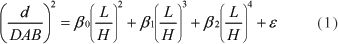where: d = diameter at height h from the soil (cm); L = H  h; bi = parameters to be estimated; e = estimation error.

Isolating d, a taper function is obtained to estimate the correspondent diameter at any height on the tree, if DAB, H and L are given.Considering the sectional area (A) of a tree with diameter d (m; at height h) equal to (p/40000) d2 and the integration of this section along length L, we obtain the compatible volume equation:Substituting (1) into (2) and integrating we obtain: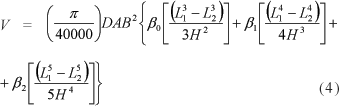Setting L2 = 0 (top of the tree) and L1 = H (base of the tree), the volume equation for the whole tree becomes: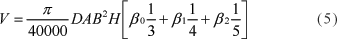and the equation used to estimate volumes at heights hi = 3, 6 and 12 m is:ii) Model 2

Polynomial model 2 (M2) can be represented, mathematically, as follows:Isolating d, we obtain the taper function to estimate the correspondent diameter at any height in the tree, if DBH, H and h are given.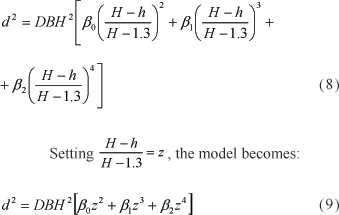As in M1, integration of section (p/40000)d2 along length L (equation 3) results in the following compatible volume equation: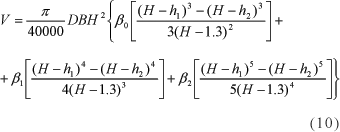Again, setting h2 = H (top) and h1 = 0 (tree base), the volume equation for the whole tree becomes:Equations used to estimate volumes at heights hi = 3, 6 and 12 m are: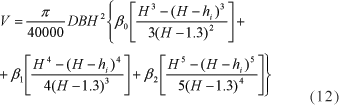Data stored formed the "base" file, which was processed by a SAS© routine to generate linear regression estimates and by LINDO© to process the goal programming model.

b i Parameter Estimation

Estimating bi parameters involves the generation of the minimum possible error (e). The minimum loss function can be defined as the differences between the observed data and the data estimated by the model.

In the ordinary least squares (OLS) method, the loss function to be minimized is set as the sum of squared residuals. Squaring the residues avoids residue canceling but weights more heavily large residues and emphasizes their importance (Batista, 1998).

In goal programming, the loss function used to estimate the bi parameters is the sum of absolute residuals and the method is referred to as the MOTAD (minimization of total absolute deviations) method. The MOTAD method also avoids residue canceling, but differently to the OLS method large residues have the same importance as small residues (Batista, 1998).

Ignizio & Cavalier (1994) discuss the use goal programming as an alternative tool for developing predictive function. According to these authors, the OLS method is more frequently employed simply because it is easy to be applied and because it generates confidence intervals based on the assumption that errors are normally distributed with equal variances, an assumption that sometimes may not hold. Alcântara et. al (2003) points out that the MOTAD method overcomes a deficiency in the OLS method when outliers are present in the data set due to MOTAD's lower sensitivity to extreme values.

Model fitting with the OLS method

The models adopted in the present study can be written as multiple linear regression models with three predictor variables, as follows: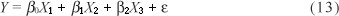where: Y = (d/DAB)2 or (d/DBH)2; X1 = (L/H)2 or [(H-h)/(H-1.3)]2; X2 = (L/H)3 or [(H-h)/(H-1.3)]3; X3 = (L/H)4 or [(H-h)/(H-1.3)]4

The condition b0 + b1 + b2 = 1 was imposed to inforce coherence. This is needed because (L/H)2, (L/H)3 and (L/H)4 are equal to 1 when L is equal to H, and also d equals to DAB, resulting consequently (d/DAB)2 equal to 1. The same reasoning can be used for M2.

Model fitting with the MOTAD method

The MOTAD method formulates the minimization of the sum of absolute deviations as a goal programming problem (GP), a mathematical formulation for constrained multiple objectives. Deviations to these objectives are minimized, generating solutions close to certain aspiration levels. Aspiration levels are in fact goals associated with the multiple objectives and, therefore, the name goal programming.

The Simplex algorithm for solving linear programming problems is normally used in the solution of GP problems. These problems can also be formulated under the same hypothesis, limitations and conditions of linear programming: linearity, divisibility and deterministic characteristic (Lee et al., 1990).

The first application of GP to constrained regression was formulated by Charnes et al. (1955). Two deviations, DPi and DNi, are created to each pair of observations (Xi,Yi). DPi represents a positive deviation and DNi represents a negative deviation. So, the linear model Yi = f(Xi) becomes 0 = f(Xi) - DPi + DNi - Yi.

In the MOTAD version of the GP problem applied to the taper function fitting process, i identifies each observation in a set of N measurements, the coefficients of the linear model are the main decision variables and the GP formulation becomes:subject to 0 = f(Xi) - DPi + DNi - Yi (i = 1, 2, ... , N)

For M1, the constraints can be represented as:and for M2, the expression becomeswhere: i = ith tree log and ith line in the model;

DNi = negative deviation of observation i in relation to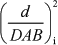or;

DPi = positive deviation of observation i in relation toor;andare goals;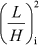,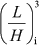,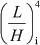,,and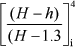are coefficients of the parameters to be estimated; and

b0, b1 and b2 are the parameters to be estimated.

Comparing the OLS and MOTAD methods

The coefficient of determination (R2), the root of the mean square error (Syx) and the dispersion of residuals were used to compare the results of the fitting process. Precision and accuracy analysis were also made based on the estimative generate by each fitting method and according to four statistics used by Parresol et al. (1987) and Assis et al. (2002): bias (), standard deviation of differences (SD), sum of squared relative residuals (SSRR) and residuals percentage (RP), where:Standard deviation of Differences (SD):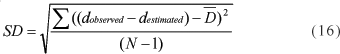Sum of Squared Relative Residuals (SSRR):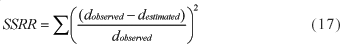Residuals percentage (RP):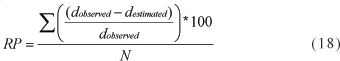Both models and the fitting methods were compared and also ranked for the quality of the estimates obtained for volume outside and inside bark (Vob and Vib). The model with the worst value for each of the calculated characteristic scored 1, otherwise the score was 2. The sum of scores for each model determined its final performance score.

RESULTS AND DISCUSSION

Model fitting

The tested models fitted adequately to data given that both models resulted in coefficients of determination above 96% (Table 1). As expected, models fitted with the MOTAD method produced coefficients of determination slightly lower than models fitted with the OLS method. The root of the mean square error ranged from 10.58% to 13.10% and resulted very similar when comparing the two fitting methods. The t and F tests were significant at 5%, showing a strong correlation between the dependent variable (d/DAB or d/DBH) and the independent variables (L/H) or [(H-h)/H-1.3)].

For diameters close to DBH and above, M2 shows residuals more clustered around zero (graphs c, d, g and h, Figure 1). Graphs (a), (b), (e) and (f) in Figure 1 show that outside bark M1 taper function with the dependent variable (d/DAB)2 represents better the form of the trunk at the base of the tree. At the base of the tree, the dependent variable (d/DBH)2 is overestimated by M2 when fitting outside bark data (graphs c and d, Figure 1) and underestimated by M2 when fitting inside bark data (graphs g and h, Figure 1).

Loss Function Minimization

Table 2 shows very small differences between the two models and the two fitting methods. Models fitted for inside bark diameters produced smaller residuals sums.

As expected, linear regression effectively resulted in the minimum sum of squared deviations while goal programming produced the minimum sum of absolute deviations.

Diameter, Vib and Vob estimation

Table 3 shows the values of the four selected statistics to evaluate the quality of estimates for diameter at different heights: bias (), standard deviation of differences (SD), sum of squared relative residuals (SSRR) and residuals percentage (RP).

The models showed to be equally precise in the estimation of diameters outside bark at relative heights equal to 3, 6 and 12 m. This could be observed for the OLS method, as well as for the MOTAD method. Considering the total height of the trees, M1 was slightly superior to M2.

Low values were observed for bias. Although low and considering the estimated diameters at 3, 6 and 12 m heights, this statistic shows that M1 tended to slightly overestimate the diameters outside and inside bark; M2 tended to slightly underestimate the diameters outside bark and overestimate the diameters inside bark; and for the top height diameters: M2 slightly overestimates inside bark while underestimating outside bark, and M1 underestimates both outside and inside.

Table 4 presents the score values for each diameter estimation model at different heights (3, 6, 12 m, and total height), for each statistic separately and for the final total score.

The statistics calculated for the volume estimation at different heights are shown in Tables 5 and 6.

The OLS and MOTAD methods fitted models 1 and 2 with similar precision. M1 showed consistently better results than M2 for inside bark volume estimation at any height. For outside bark, M1 resulted better only for heights 3 and 6 m. For volumes up to 12 m, both fitting models resulted similar, and for total tree outside bark volume M2 showed more precise.

Both polynomial functions fitted well the data along the main part of the tree except for the base part of the trunk. Differences between the two fitting methods, Ordinary Least Squares (OLS) and Minimization of Total Absolute Deviations (MOTAD), were practically insignificant in terms of generating good estimates for the taper function coefficients. Weights to reduce heteroscedasticity were not considered in this study, and are strongly recommended on further analysis of the two fitting methods in the future. Although rarely used on practical forest assessments, the diameter at the base of the tree (DAB) was here considered to create the opportunity to evaluate the sensitivity of the MOTAD fitting method to situations where the variance highly increases when measurement values also increase.

ACKNOWLEDGEMENTS

To Antonilmar A. Lopes da Silva and João Fernando Borges, Veracel Celulose S.A., for the supply of the data and authorizing their publication.

Accepted July 21, 2006

• AHRENS, S.; HOLBERT, D. Uma função para forma de tronco e volume de Pinus taeda L Curitiba: EMBRAPA, 1981. p.37-68. (Boletim de Pesquisa Florestal, 3).
• ALCÂNTARA, A.A.M.; SANT'ANNA, A.P.; LINS, M.P.E. Restringindo flexibilidade de pesos em DEA utilizando análise de regressão MSEA. Pesquisa Operacional, v.23, p.347-357, 2003.
• ANDRADE, V.C.L. de; LEITE, H.G. Um método para quantificar multiprodutos de árvores individuais na unidade estere. Revista Árvore, v.22, p.299-306, 1998.
• ASSIS, A.L. de. Avaliação de modelos polinomiais segmentados e não-segmentados na estimativa de diâmetro e volumes comerciais de Pinus taeda Lavras: UFLA, 2000. 189p.
• ASSIS, A.L.; SCOLFORO, J.R.S.; MELLO, J.M. de; OLIVEIRA, A.D. de. Avaliação de modelos polinomiais segmentados e não-segmentados na estimativa de diâmetro e volumes comerciais de Pinus taeda. Ciência Florestal, v.12, p.89-107, 2002.
• BATISTA, J.L.F. Análise de regressão: técnicas de modelagem florestal. Piracicaba: ESALQ, 1998. 12p. (Apostila da disciplina Dendrometria).
• CHARNES, A.; COOPER, W.W.; FERGUSON, R. Optimal estimation of executive compensation by goal programming. Management Science, v.1, p.138-151, 1955.
• CLUTTER, J. L. Development of taper functions from variable-top merchantable volume equations. Forest Science, v. 26, p.117-120, 1980.
• DEMAERSCHALK, J. P. Integrated systems for the estimation of tree taper and volume. Canadian Journal of Forest Research, v. 3, p.90-94, 1973.
• FISCHER, F.E. Eficiência dos modelos polinomiais e das razões de volume na estimativa volumétrica dos sortimentos e do perfil do fuste de Pinus taeda Lavras: UFLA, 1997. 167p. (Dissertação Mestrado em Engenharia Florestal).
• GUIMARÃES, D.P.; LEITE, H.G. Um novo modelo para descrever o perfil do tronco. Revista Árvore, v.16, p.170-180, 1992.
• HUSCH, B.; MILLER, C.I.; BEERS, T.W. Forest mensuration 2.ed. New York: The Ronald Press, 1972. 410p.
• IGNIZIO, J.P.; CAVALIER, T.M. Linear programming Englewood Cliffs: Prentice Hall, 1994. 666p.
• KOZAK, A.; MUNRO, D.D.; SMITH, J.H.G. Taper functions and their application in forest inventory. Forest Chronicle, v.45, p.278-283, 1969.
• LEE, S.M.; MOORE, L.J.; TAYLOR, B.W. Management science 3.ed. Boston: Allyn & Bacon, 1990. cap.13, p.657-718: Goal programming.
• LEITE, H. G.; GUIMARÃES, D. P.; CAMPOS, J. C. C. Descrição e emprego de um modelo para estimar múltiplos volumes de árvores. Revista Árvore, v.19, p.65-79, 1995.
• LIMA, F.S. Análise de funções de "taper" destinadas à avaliação de multiprodutos de árvores de Pinus elliottii Viçosa: UFV, 1986. 79p.
• MAX, T.A.; BURKHART, H.E. Segmented polynomial regressions applied to taper equations. Forest Science, v.22, p.283-289, 1976.
• MCTAGUE, J.P.; BATISTA, J.L.F.; STEINER, L.H. Equações de volume total, volume comercial e forma do tronco para plantações de Eucalyptus nos estados de São Paulo e Rio de Janeiro. IPEF, n.41/42, p.56-63, 1989.
• ORMEROD, D.W. A simple bole model. Forestry Chronicle, v.49, p.136-138, 1973.
• PARRESOL, B.R; HOTVEDT, J.E.; CAO, Q.V. A volume and taper prediction system for bald cypress. Canadian Journal of Forest Research, v.17, p.250-259, 1987.
• SCHNEIDER, P.R.; FINGER, C.A.G.; KLEIN, J.E.M.; TOTTI, J.A.; BAZZO, J.L. Forma de tronco e sortimentos de madeira de Eucalyptus grandis Maiden para o Estado do Rio Grande do Sul. Ciência Florestal, v.6, p.79-88, 1996.
• SCOLFORO, J.R. Mensuração florestal: relações quantitativas em volume, peso e a relação hipsométrica. Lavras: ESAL/FAEPE, 1993. 298p.
• *
Corresponding author <

# Publication Dates

• Publication in this collection
20 Sept 2006
• Date of issue
Oct 2006